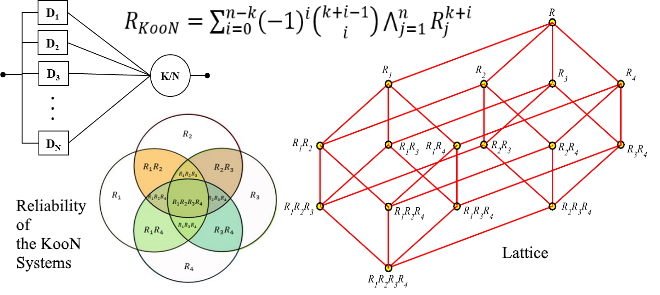# A Simple Sum of Products Formula to Compute the Reliability of the KooN SystemReliability block diagram (RBD) is a well-known, high-level abstract modeling method for calculating systems reliability. Increasing redundancy is the most important way for increasing Fault-tolerance and reliability of dependable systems. K-out-of-N (KooN) is one of the known redundancy models. The redundancy causes repeated events and increases the complexity of the computing system’s reliability, and researchers use techniques like factorization to overcome it. Current methods lead to the cumbersome formula that needs a lot of simplification to change in the form of Sum of the Products (SoP) in terms of reliabilities of its constituting components. In This paper, a technique for extracting simple formula for calculating the KooN system’s reliability in SoP form using the Venn diagram is presented. Then, the shortcoming of using the Venn diagram that is masking some joints events in the case of a large number of independent components is explained. We proposed the replacement of Lattice instead of Venn diagrams to overcome this weakness. Then, the Lattice of reliabilities that is dual of power set Lattice of components is introduced. Using the basic properties of Lattice of reliabilities and their inclusion relationships, we propose an algorithm for driving a general formula of the KooN system’s reliability in SoP form. The proposed algorithm gives the SoP formula coefficients by computing elements of the main diagonal and elements below it in a squared matrix. The computational and space complexity of the proposed algorithm is θ ((n – k) 2 /2) that n is the number of different components and k denotes the number of functioning components. A lemma and a theorem are defined and proved as a basis of the proposed general formula for computing coefficients of the SoP formula of the KooN system. Computational and space complexity of computing all of the coefficients of reliability formula of KooN system using this formula reduced to $\theta (n-k)$ . The proposed formula is simple and is in the form of SoP, and its computation is less error-prone.